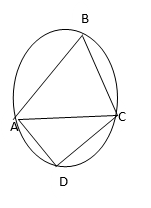### Chord Properties Continued

Mathematics SSS 2 Second Term

WEEK 8

Chord Properties Continued

Performance Objectives

Students should be able to;

1. Solve problems on angles subtended by two equal chords at the centre
2. Solve Problems on perpendicular bisectors of chord
3. Solve Problems on angles in the alternate segment

Angles suspended by two equal chords at the centre

Content

An inscribed angle has its vertex on the circle. ABC, in the diagram below, is called an inscribed angle or angle at the circumference. The angle is also said to be subtended by (i.e. opposite to) arc ADC or chord AC